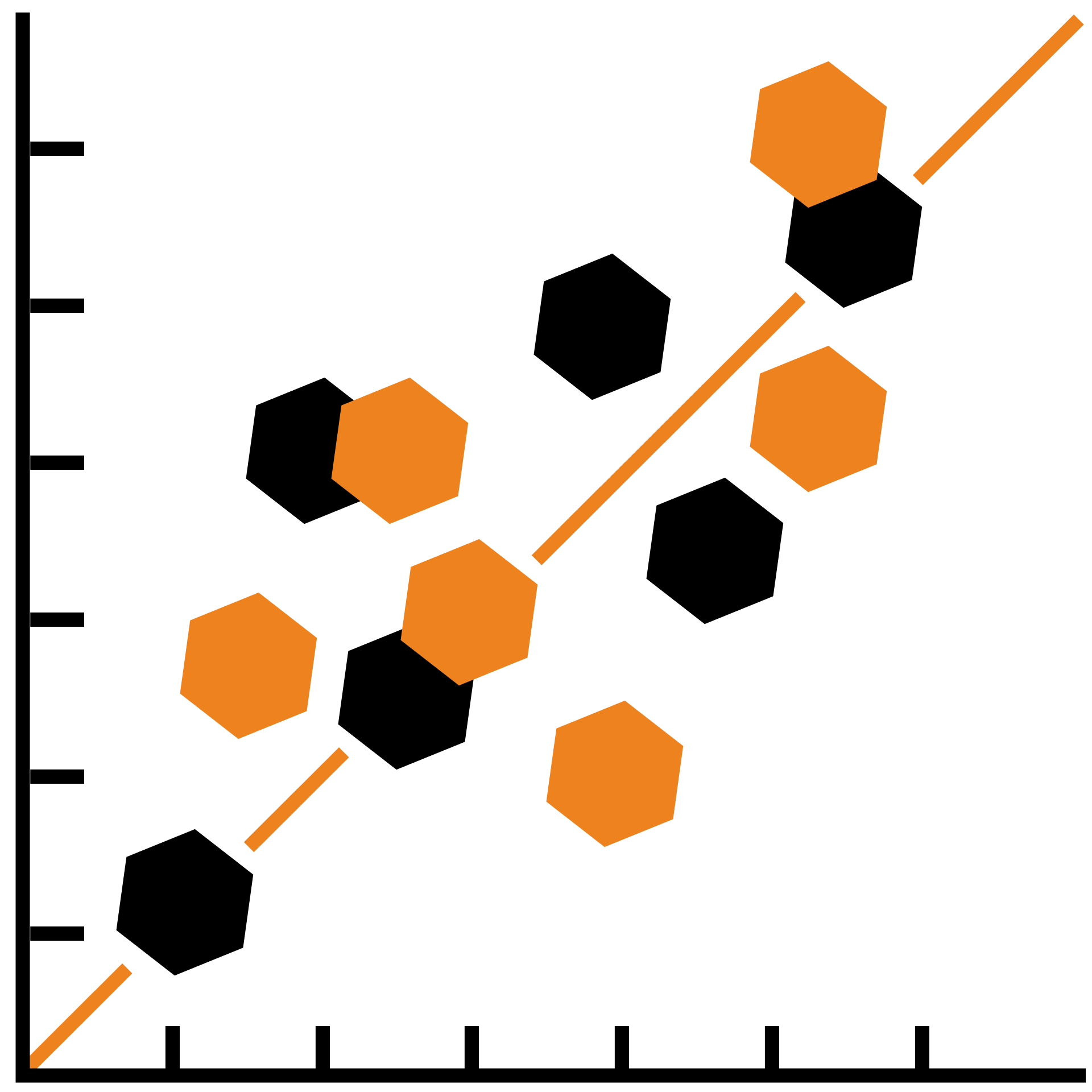## Statistics Fundamentals

Data can be deceiving. Learn to use math to discern truth from fiction.

Riddles on Averages

Solving Forward, Solving Backward

Deception

Mean and Median

Mode

Quantiles

IQR

A Probability Refresher

Scatterplots and Regression

Bar and Line Graphs

More Graphs

Cumulative Frequency Plots

Variance and Standard Deviation

Building the Normal Curve

Mathematical Bias

The n-1 Mystery

Observation vs. Experiment

Bayesian Probability

Experiment Design

Blocking

Confidence Intervals

Hypothesis Testing

Type I and Type II Errors

### Course description

Statistics starts from data and then asks what was used to generate it. Learning the building blocks — median, mode, range, variance, and standard deviation — will help you analyze graphs, determine statistical significance, and make informed decisions. By the end of this course, you'll be able to mathematically quantify predictions, use statistical tools to conduct experiments, and discern the truth in a set of data.

### Topics covered

• Arithmetic Mean
• Median
• Mode
• Quantiles
• IQR
• Variance
• Standard Deviation
• Regression
• Deceptive Graph Design
• Cumulative Frequency Plots
• p-values
• Normal Curves
• Experiment Design
• Type I and Type II errors